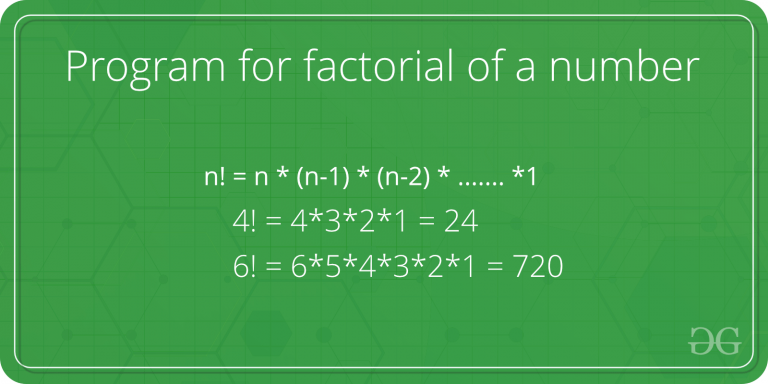# Scala program to find Factorial of a number

Given a number N, the task is to calculate factorial of N.

In mathematics, the factorial of a positive integer N is the product of all positive integers less than or equal to N. The recursive formula to calculate factorial of a given positive integer N is

```N! = N * ( N -1 )!
N! = 1 if N = 1 or N = 0
```Examples:

```Input : N = 3
Output : 6

Input : N = 5
Output : 120
```

## Recommended: Please try your approach on {IDE} first, before moving on to the solution.

Method 1 : Iterative Way
In this method, we will use a loop to iterate over the sequence of numbers to get the factorial.
Below is the implementation of above approach
Example :

 `// Scala Program to calculate ` `// Factorial of a number ` ` `  `// Creating object ` `object` `GFG ` `{ ` `    ``// Iterative way to calculate ` `    ``// factorial  ` `    ``def` `factorial(n``:` `Int)``:` `Int ``=` `{ ` `         `  `        ``var` `f ``=` `1` `        ``for``(i ``<``- ``1` `to n) ` `        ``{ ` `            ``f ``=` `f * i; ` `        ``} ` `         `  `        ``return` `f ` `    ``} ` ` `  `    ``// Driver Code ` `    ``def` `main(args``:` `Array[String])  ` `    ``{ ` `        ``println(factorial(``5``)) ` `    ``} ` ` `  `} `

Output :

```120
```

Method 2 : Use of Recursion
In this method, Recursive formula N! = N * (N -1) ! is used to calculate the factorial of the given number.
Below is the implementation of above approach.
Example :

 `// Scala Program to calculate Factorial ` `// of a number using recursion ` ` `  `// Creating object ` `object` `GFG ` `{ ` `    ``// Function to calculate ` `    ``// factorial using Recursive  ` `    ``// formula (i.e N! = N * N-1 !) ` `    ``def` `factorial(n``:` `Int)``:` `Int ``=` `    ``{ ` `        ``if` `(n ``==` `0``)  ` `            ``return` `1` `        ``else` `            ``return` `n * factorial(n-``1``) ` `    ``} ` ` `  `    ``// Driver Code ` `    ``def` `main(args``:` `Array[String])  ` `    ``{ ` `        ``println(factorial(``5``)) ` `    ``} ` `} `

Output :

```120
```

My Personal Notes arrow_drop_upself motivated and passionate programmer

If you like GeeksforGeeks and would like to contribute, you can also write an article using contribute.geeksforgeeks.org or mail your article to contribute@geeksforgeeks.org. See your article appearing on the GeeksforGeeks main page and help other Geeks.

Please Improve this article if you find anything incorrect by clicking on the "Improve Article" button below.

Article Tags :

Be the First to upvote.

Please write to us at contribute@geeksforgeeks.org to report any issue with the above content.